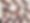# 7-Eleven

\$6 Delivery
1717 East Pflugerville Parkway

\$3.79
+

\$3.19
+

\$3.29
+

\$5.99
+

\$1.89
+

\$2.99
+

\$4.79
+

\$1.89
+

\$1.19
+

\$2.19
+

\$1.99
+

\$1.39
+

\$1.89
+

\$1.69
+

\$3.59
+

\$3.29
+

\$1.79
+

\$2.09
+

\$3.09
+

\$2.59
+

\$2.09
+

\$2.59
+

\$1.89
+

\$1.49
+

\$4.39
+

\$2.39
+

\$4.79
+

\$2.99
+

\$2.99
+

\$7.19
+

\$3.49
+

\$3.89
+

\$2.09
+

\$2.39
+

\$1.79
+

\$2.39
+

\$2.09
+

\$2.19
+

\$1.79
+

\$2.19
+

\$3.59
+

\$2.59
+

\$2.59
+

\$1.19
+

\$2.09
+

\$2.39
+

\$4.29
+

\$2.39
+

\$2.39
+

\$2.39
+

\$2.39
+

\$2.59
+

\$2.59
+

\$1.99

15 count

+

\$2.39
+

\$2.39
+

\$1.79
+

\$1.79
+

\$1.79
+

\$1.79
+

\$7.00
+

\$7.00
+

\$1.79
+

\$5.00

(5 piece)

+

\$5.00

(5 piece)

+

\$2.39
+

\$3.59
+

\$3.59
+

\$3.99
+

\$4.59
+

\$3.99
+

\$3.99
+

\$2.39
+

\$2.59

4 oz

+

\$5.00

(5 piece)

+

\$1.29
+

\$2.39
+

\$3.89
+

\$4.99
+

\$4.99
+

\$2.99

6"

+

\$2.79
+

\$1.59

10 oz

+

\$1.00
+

\$2.39
+

\$5.00

20 pcs

+

\$1.99
+

\$6.99
+

\$7.79
+

\$4.39
+

\$6.89
+

\$6.89
+

\$6.89
+

\$3.99
+

\$3.99
+

\$3.99
+

\$3.99
+

\$3.99
+

\$3.99
+

\$3.99
+

\$3.99
+

\$3.99
+

\$3.99
+

\$4.59
+

\$7.09
+

\$3.09
+

\$4.39
+

\$3.89
+

\$2.39
+

\$5.39
+

\$4.49
+

\$1.79
+

\$1.79
+

\$1.19
+

\$1.19
+

\$2.69
+

\$1.19
+

\$1.19
+

\$1.19
+

\$1.19
+

\$1.99
+

\$5.39
+

\$3.09
+

\$4.29
+

\$3.29
+

\$4.79
+

\$1.99
+

\$2.69
+

\$1.99
+

\$3.29
+

\$2.39
+

\$8.39
+

\$3.59
+

\$3.59
+

\$2.69
+

\$4.29
+

\$2.39
+

\$2.69
+

\$2.69
+

\$1.99
+

\$4.69
+

\$4.99
+

\$2.69
+

\$1.99
+

\$1.19
+

\$7.09
+

\$7.49
+

\$11.69
+

\$3.39
+

\$11.69
+

\$10.79
+

\$7.49
+

\$7.79
+

\$2.99
+

\$5.39
+

\$2.39
+

\$5.59
+

\$4.79
+

\$4.19
+

\$5.29
+

\$7.49
+

\$4.79
+

\$6.59
+

\$7.19
+

\$7.19
+
9. ## House Hold

\$3.09
+

\$8.99
+

\$8.99
+

\$3.59
+

\$3.69
+

\$2.09
+

\$2.69
+

\$5.99
+

\$4.39
+

\$3.59
+

\$2.39
+

\$4.79
+

\$5.29
+

\$4.79
+

\$2.99
+

\$3.59
+

\$7.19
+

\$3.59
+
19. ### 7-Select Glass Cleaner

\$4.79
+
10. + Add a Custom Item